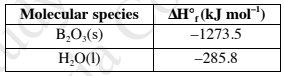# Problem: Pentaborane B5H9(s) burns vigorously in O2 to give B2O3(s) and H2O(l):2 B5H9(s) + 12 O2(g) ⟶5 B2O3(s) + 9 H2O(l)The standard enthalpy change for this reaction is -9086.1 kJ. Given the following data, determine the standard enthalpy of formation for B5H9.A. -1273.5 kJ mol-1  B. -846.1 kJ mol-1  C. 73.2 kJ mol-1  D. -2547.0 kJ mol-1  E. 146.4 kJ mol-1

###### FREE Expert Solution

We’re being asked to determine the standard enthalpy formation (ΔH˚f) for B5H9

Recall that ΔH˚rxn can be calculated from the enthalpy of formation (ΔH˚f) of the reactants and products involved

95% (300 ratings)###### Problem Details

Pentaborane B5H9(s) burns vigorously in O2 to give B2O3(s) and H2O(l):

2 B5H9(s) + 12 O2(g) ⟶5 B2O3(s) + 9 H2O(l)

The standard enthalpy change for this reaction is -9086.1 kJ. Given the following data, determine the standard enthalpy of formation for B5H9.A. -1273.5 kJ mol-1

B. -846.1 kJ mol-1

C. 73.2 kJ mol-1

D. -2547.0 kJ mol-1

E. 146.4 kJ mol-1